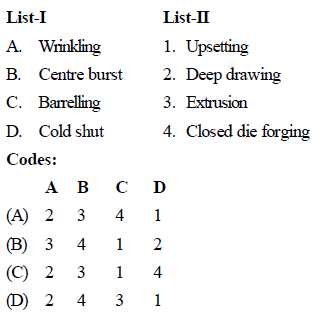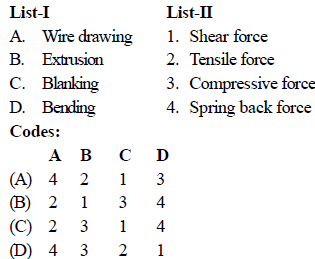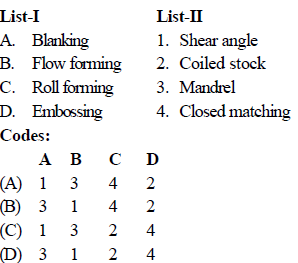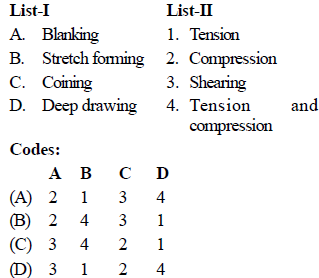Courses

# Test: Production Test (Level - II)- 2

## 25 Questions MCQ Test Mechanical Engineering SSC JE (Technical) | Test: Production Test (Level - II)- 2

Description
This mock test of Test: Production Test (Level - II)- 2 for Mechanical Engineering helps you for every Mechanical Engineering entrance exam. This contains 25 Multiple Choice Questions for Mechanical Engineering Test: Production Test (Level - II)- 2 (mcq) to study with solutions a complete question bank. The solved questions answers in this Test: Production Test (Level - II)- 2 quiz give you a good mix of easy questions and tough questions. Mechanical Engineering students definitely take this Test: Production Test (Level - II)- 2 exercise for a better result in the exam. You can find other Test: Production Test (Level - II)- 2 extra questions, long questions & short questions for Mechanical Engineering on EduRev as well by searching above.
QUESTION: 1

Solution:
QUESTION: 2

Solution:
QUESTION: 3

### Swaging is an operation of

Solution:
QUESTION: 4

Bolt heads are manufactured by

Solution:
QUESTION: 5

In the forging operation, fullering is done to

Solution:
QUESTION: 6

The process of coining is

Solution:
QUESTION: 7

Which of the following manufacturing process will produce strongest component ?

Solution:
QUESTION: 8

Which of the following metals is best suitable for extrusion either hot or cold ?

Solution:
QUESTION: 9

What does hydrostatic pressure in extrusion process improve ?

Solution:
QUESTION: 10

A floating mandrel is used in

Solution:
QUESTION: 11

Operation of producing number of holes evenly spaced in a regular pattern on a sheet of metal is known as

Solution:
QUESTION: 12

Spring back factor of the sheet metal depends on

Solution:
QUESTION: 13

Mechanical property that decides the cutting force in the blanking operation is

Solution:
QUESTION: 14

In a four-high rolling mill stand, the diameter of  backing up rolls in compression to diameter of working roll is

Solution:
QUESTION: 15

In rolling a strip between two rolls, the position of the neutral point in the arc of contact does not depend on

Solution:
QUESTION: 16

In the rolling process, roll separating force can be decreased by

Solution:
QUESTION: 17

Match List-I with List-II and select the correct answer using the codes given below the lists :Solution:
QUESTION: 18

Cold shut is a forging defect caused by which of the following reason ?

Solution:
QUESTION: 19

In drawing operation if Di = initial diameter and D0 = outgoing diameter, then the degree of drawing equal to ?

Solution:
QUESTION: 20

Which of the following material is used in manufacturing of extrusion nozzles ?

Solution:
QUESTION: 21

Match List-I (Metal forming process) with List-II (Associated force) and select the correct answer using the codes given below the lists :Solution:
QUESTION: 22

Match List-I (Metal forming process) with List-II (Associated feature) and select the correct answer using the codes given below the lists:Solution:
QUESTION: 23

Match List-I (Operations) with List-II (Stresses induced) and select the correct answer using the codes given below the lists :Solution:
QUESTION: 24

The products made by powder metallurgy process are very strong in

Solution:
QUESTION: 25

Sintering time is minimum for

Solution: